Processing ......FreeComputerBooks.com Links to Free Computer, Mathematics, Technical Books all over the World

Computational and Mathematical Simulations and Modeling. Monte Carlo Method
Related Book Categories:
•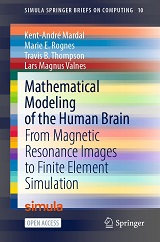Mathematical Modeling of the Human Brain

The book bridges common tools in medical imaging and neuroscience with the numerical solution of brain modelling PDEs, covers the basics of magnetic resonance imaging and quickly proceed to generating first FEniCS brain meshes from T1-weighted images.

•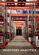Inventory Analytics: A Practicable, Python-Driven Approach

This book provides a comprehensive and accessible introduction to the theory and practice of Inventory Control – a significant research area central to supply chain planning. It adopts a practicable, Python-driven approach to illustrating theories and concepts.

•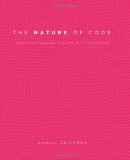The Nature of Code: Simulating Natural Systems with Processing

A range of programming strategies and techniques behind computer simulations of natural systems, from elementary concepts in mathematics and physics to more advanced algorithms that enable sophisticated visual results, using Processing.

•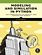Modeling and Simulation in Python (Allen B. Downey)

This book is an introduction to physical modeling using a computational approach with Python. You will learn how to use Python to accomplish many common scientific computing tasks: importing, exporting, and visualizing data; numerical analysis; etc.

•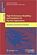Modelling and Simulation for Big Data Applications

Long considered important pillars of the scientific method, Modelling and Simulation have evolved from traditional discrete numerical methods to complex data-intensive continuous analytical optimisations.

•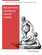High Performance Computing and Numerical Modelling

This textbook provides a step-by-step approach to numerical methods in engineering modelling. It provides a consistent treatment of the topic, from the ground up, to reinforce for students that numerical methods are a set of mathematical modelling tools.

•A Gentle Introduction to Numerical Simulations with Python

This book outlines the shortest possible path from no previous experience with programming to a set of skills that allows students to write simple programs for solving common mathematical problems with numerical methods in the context.

•Modeling Creativity - Case Studies in Python (Tom De Smedt)

This book is to model creativity using computational approaches in Python. The aim is to construct computer models that exhibit creativity in an artistic context, that is, that are capable of generating or evaluating an artwork (visual or linguistic), etc.

•Computer Simulation Techniques - The Definitive Introduction

This book addresses all the important aspects of a computer simulation study, including modeling, simulation languages, validation, input probability distribution, and analysis of simulation output data.

•Learning Processing: Guide to Programming Images, Animation

This book teaches you the basic building blocks of programming needed to create cutting-edge graphics applications including interactive art, live video processing, and data visualization, using Processing..

•Numerical Simulations of Physical and Engineering Processes

The book handles the numerical simulations of physical and engineering systems. It can be treated as a bridge linking various numerical approaches of two closely inter-related branches of science, i.e. physics and engineering.

•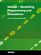MATLAB - Modelling, Programming and Simulations (E. P. Leite)

This is an authoritative guide to generating readable, compact, and verifiably correct MATLAB programs. It is ideal for undergraduate engineering courses in Mechanical, Aeronautical, Civil, and Electrical engineering that require/use MATLAB.

•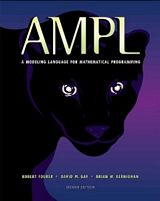AMPL: A Modeling Language for Mathematical Programming

This book is a complete guide to AMPL for modelers at all levels of experience. It begins with a tutorial on widely used linear programming models, and presents all of AMPL's features for linear programming with extensive examples.

•Modeling Reactive Systems With Statecharts (David Harel)

The book provides a detailed description of a set of languages for modelling reactive systems, which underlies the STATEMATE toolset. The approach is dominated by the language of Statecharts, used to describe behavior and activities.

•Computational and Numerical Simulations (Jan Awrejcewicz)

It presents both new theories and their applications, showing bridge between theoretical investigations and possibility to apply them by engineers of science, handles the recent research devoted to numerical simulations of physical and engineering systems.

•Theory and Applications of Monte Carlo Simulations (Wai Kin Chan)

The purpose of this book is to introduce researchers and practitioners to recent advances and applications of Monte Carlo Simulation (MCS). Random sampling is the key of the MCS technique.

•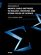Applications of Monte Carlo Methods in Biology, Medicine, etc.

This book focuses on the state of the art of Monte Carlo methods Applications of Monte Carlo Methods in Biology, Medicine and Other Fields of Scienc. The main thrust of this book is to emphasize applications in biology and medicine.

•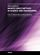Applications of Monte Carlo Method in Science and Engineering

This book exposes the broad range of applications of Monte Carlo simulation in the fields of Quantum Physics, Statistical Physics, Reliability, Medical Physics, Polycrystalline Materials, Ising Model, Chemistry, Agriculture, Food Processing, X-ray Imaging, ...

•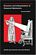Structure and Interpretation of Classical Mechanics

This innovative textbook concentrates on developing general methods for studying the behavior of classical systems. It focuses on the phenomenon of motion and makes extensive use of computer simulation in its explorations of the topic.

•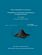From Algorithms to Z-Scores: Probabilistic and Statistical Modeling

This is a textbook for a course in mathematical probability and statistics for computer science students.

•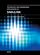Technology and Engineering Applications of SIMULINK

Present the procedural steps required for modeling and simulating the basic dynamic system problems in SIMULINK, built on the top of MATLAB to provide a platform for engineers to plan, model, design, simulate, test and implement complex systems.

•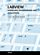Modeling, Programming and Simulations Using LabVIEW™ Software

In this book a collection of applications covering a wide range of possibilities is presented. We go from simple or distributed control software to modeling done in LabVIEW to very specific applications to usage in the educational environment.

•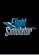Flight Simulation Books (Kevin Savetz, et al)

A collection of 21 Classical Flight Simulation Books available on the web. Everything there is available with permission of the copyright holders. More than 2,200 printed pages have been digitized into 800+ web pages.

Book Categories
 :All CategoriesRecent BooksMiscellaneous BooksComputer LanguagesComputer ScienceData Science/DatabasesElectrical EngineeringJava and Java EE (J2EE)Linux and UnixMathematicsMicrosoft and .NETMobile ComputingNetworking and CommunicationsSoftware EngineeringSpecial TopicsWeb Programming
Other Categories